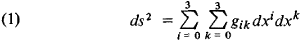# Space-Time Metric

## Space-Time Metric

a metric that determines the geometric properties of four-dimensional space-time (which connects physical three-dimensional space and time) in relativity. A space-time metric is characterized by an invariant quantity (a quantity independent of the frame of reference)—the square of the four-dimensional interval—which defines the space-time connection (the square of the “distance”) between two infinitesimally close events,Here dx1, dx2, and dx3 are the differences in the spatial coordinates of the events, dx0 = cdt, where dt is the time difference between these events, c is the velocity of light, and the#/* are the components of the metric tensor. In the general case, the metric tensor satisfies Einstein’s equations of general relativity, and the components gn are functions of the coordinates x1, x2, x3, and x0. The form of these functions in the selected frame of reference depends on the masses present in space-time. In the absence of large masses, the metric tensor can be reduced to the form

(2) g11 = g22 = g33 = −1, g00 = +1, gik = 0 when ik

Then, in rectangular Cartesian coordinates, with x1 = x, x2 = y, and x3 = z,we obtain

(3) ds2 = c2dtdx2dy2dz2

A space-time with such a metric is a Euclidean space (or, more accurately,a pseudo-Euclidean space, because of the minus sign preceding dx2, dy2, and dz2); it is called “flat space.” Such a space-time metric—or the equivalent metric of Minkowski space —arises in special relativity.

If large masses are present, the metric tensor cannot be reduced by any coordinate transformation to the form (2) throughout all space-time. This means that space-time is curved and its curvature is defined by the components gik (and their derivatives with respect to the coordinates). Thus the geometric properties of space-time (its metric) depend on the matter present in it. The degree of deviation of a space-time metric from a Euclidean metric is determined by the distribution of the masses in the space and by their motion. The gravitational field that is due to the masses and that, in turn, causes the motion of the masses is viewed in general relativity as a manifestation of the curvature of space-time; the gravitational field, just like the space-time metric, is determined by the values of the gv*. The curvature of space-time denotes, in particular, both the deviation of purely spatial geometry from Euclidean geometry and also the dependence of the rate of flow of time on the gravitational field.

G. A. ZISMAN

References in periodicals archive ?
The reader will see that the kinematic condition (1) on the space-time metric plays an important role in the induced geometry of null hypersurfaces of [bar.
Back reaction is the influence of created particles on the curved space-time metric which creates them.
In this article, the factor by which the radius of the Sun and oblate spheroidal planets is reduced to form a black hole is computed using the oblate spheroidal space-time metric.
8) However, a detailed explanation of why the relevant space-time metric in the vicinity of the earth is static (that is, unchanging over time and unchanged by reversing the direction of increasing time coordinate) and how static character entails terrestrial energy conservation, though available in the GTR literature, remains to be applied explicitly in this context.
In , Jumale (2006) has solved the field equations in non-symmetric unified field theory proposed by Einstein and obtained five dimensional (z-t)-type plane wave solutions on the lines of Takeno (1961)  with the space-time metric of Ambatkar (2002).
The speed of thought: Investigation of a complex space-time metric to describe psychic phenomena.
But in our case we have to deal with a space-time metric on R x [R.
First, it is necessary to obtain the exact solution of the Einstein field equations for the space-time metric induced by the gravitational field of a sphere of incompressible liquid.
On the ramifications of the Schwarzschild space-time metric.
1, 68-73; On the ramification of the Schwarzschild space-time metric.
From the mathematical viewpoint these forces are different, because they are included in different terms of the space-time metric.
2] becomes sign-alternating so that the space-time metric has the signature (+-+-) (this case is outside the initial statement of General Relativity).

Site: Follow: Share:
Open / Close